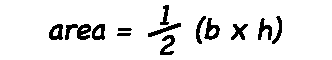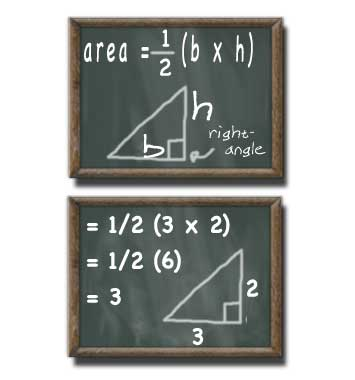# Right-Triangle Area Calculator

### Kyle's Converter > Calculators > Geometry > Right Triangle Area

For triangles without a right angle use Heron's Formula Calculator

## Right-Triangle Area

#### For Right-Angle Triangles

Result:
0.5base (b) units
height (h) decimals

A right-angle triangle with a base of 1 units and a height of 1 units has an area of 0.5 square units.

This calculator and more easy to use calculators waiting at www.KylesCalculators.com### Calculating the Area of a Right-Angle Triangle:

The area of a right-angle triangle can be calculated according to the following formula:

A = 1/2(bh)

In plain english the area of a right angle triangle can be calculated by taking one half of the base multiplied by the height.

Below is an example of how to find the area of a right-angle triangle with a base of 6 meters and a height of 3 meters.

• A = 1/2(bh)
• = 1/2(6 * 3)
• = 1/2(18)
• = 9